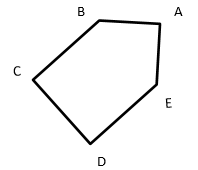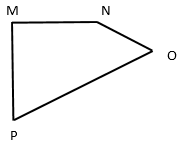# Shapes and Angles

## Objective

Identify and draw perpendicular lines.

## Common Core Standards

### Core Standards

?

• 4.G.A.1 — Draw points, lines, line segments, rays, angles (right, acute, obtuse), and perpendicular and parallel lines. Identify these in two-dimensional figures.

?

• 3.G.A.1

## Criteria for Success

?

1. Understand that intersecting lines are lines that have a point in common (i.e., lines that cross).
2. Understand that perpendicular lines are lines that intersect to form right angles.
3. Determine whether two lines are perpendicular by using the student-created right-angle template.
4. Draw perpendicular lines using a straightedge and the right-angle template (MP.5, MP.6).
5. Use correct notation to denote perpendicular lines, e.g., $\overline{AB}\perp\overline{CD}$ and draw right angle symbols in a figure.

## Tips for Teachers

?

• The following material is needed for today's lesson: Right-angle template
• Right-angle templates were constructed in Lesson 2 Anchor Task #1 from blank paper.

#### Remote Learning Guidance

If you need to adapt or shorten this lesson for remote learning, we suggest prioritizing Anchor Task 1 (benefits from worked example). Find more guidance on adapting our math curriculum for remote learning here.

#### Fishtank Plus

• Problem Set
• Student Handout Editor
• Vocabulary Package

?

### Problem 1

Use the following image to complete Parts (a)—(c) below.1. Estimate to draw point $X$ halfway up $\overline{AB}$.
2. Estimate to draw point $Y$ halfway up $\overline{CD}$.
3. Draw horizontal line segment $\overline{XY}$.  What word do the segments create? What type of angles did you create?

#### References

EngageNY Mathematics Grade 4 Mathematics > Module 4 > Topic A > Lesson 3Application Problem

Grade 4 Mathematics > Module 4 > Topic A > Lesson 3 of the New York State Common Core Mathematics Curriculum from EngageNY and Great Minds. © 2015 Great Minds. Licensed by EngageNY of the New York State Education Department under the CC BY-NC-SA 3.0 US license. Accessed Dec. 2, 2016, 5:15 p.m..

Modified by The Match Foundation, Inc.

### Problem 2

Identify perpendicular lines around the classroom and use the right-angle template to verify.

#### References

EngageNY Mathematics Grade 4 Mathematics > Module 4 > Topic A > Lesson 3Concept Development Problem 2

Grade 4 Mathematics > Module 4 > Topic A > Lesson 3 of the New York State Common Core Mathematics Curriculum from EngageNY and Great Minds. © 2015 Great Minds. Licensed by EngageNY of the New York State Education Department under the CC BY-NC-SA 3.0 US license. Accessed Dec. 2, 2016, 5:15 p.m..

Modified by The Match Foundation, Inc.

### Problem 3

Using your right-angle template, draw perpendicular line segments.

#### References

EngageNY Mathematics Grade 4 Mathematics > Module 4 > Topic A > Lesson 3Concept Development, Problem #4

Grade 4 Mathematics > Module 4 > Topic A > Lesson 3 of the New York State Common Core Mathematics Curriculum from EngageNY and Great Minds. © 2015 Great Minds. Licensed by EngageNY of the New York State Education Department under the CC BY-NC-SA 3.0 US license. Accessed Dec. 2, 2016, 5:15 p.m..

Modified by The Match Foundation, Inc.

## Problem Set & Homework

#### Discussion of Problem Set

• How did your knowledge of right angles prepare you to identify perpendicular lines in the figures for #1?
• Look at #2. How can you tell if two lines are perpendicular?
• In #4, which figures had no perpendicular lines? Explain.
• In #6, I only located eight right angles (on the interior of the figure). How many more right angles are there? What did this problem show you about locating angles on figures?
• How are perpendicular lines related to right angles? Acute angles? Obtuse angles?
• How might you use your understanding of perpendicular lines to solve a problem in real life? How might you use perpendicular lines when building something, for example?
• As you search for lines in your environment, notice if you find perpendicular or intersecting lines in nature. Analyze upright perpendicular lines, diagonal perpendicular lines, and intersecting lines as used by human beings.

?

### Problem 1

For each figure, fill in the blank to make the statement true.

a.                                                                     ${\overline{AB}\perp}$  ______b.                                                                     ${\overline{MN}\perp}$  ______#### References

EngageNY Mathematics Grade 4 Mathematics > Module 4 > Topic A > Lesson 3Exit Ticket, Question #1 and #2

Grade 4 Mathematics > Module 4 > Topic A > Lesson 3 of the New York State Common Core Mathematics Curriculum from EngageNY and Great Minds. © 2015 Great Minds. Licensed by EngageNY of the New York State Education Department under the CC BY-NC-SA 3.0 US license. Accessed Dec. 2, 2016, 5:15 p.m..

Modified by The Match Foundation, Inc.

### Problem 2

Draw an additional example of perpendicular line segments below.

### Mastery Response

?